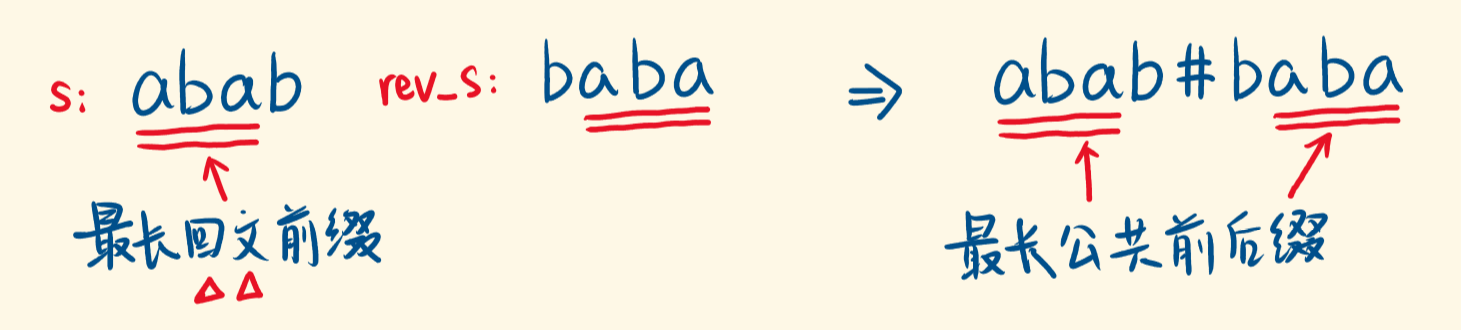# 214. 最短回文串

输入：s = "aacecaaa"



输入：s = "abcd"





0 <= s.length <= 5 * 10^4

s 仅由小写英文字母组成



# 基础

java
/**
* 是否为回文
*
* @param string 字符串
* @return 是否
*/
public static boolean isPalindrome(final String string) {
if (string.length() == 1) {
return false;
}
final int length = string.length();
int mid = length >> 1;
for (int i = 0; i < mid; i++) {
if (string.charAt(i) != string.charAt(length - i - 1)) {
return false;
}
}
return true;
}


# v1-翻转暴力法

## java 实现

class Solution {

public String shortestPalindrome(String s) {
// 本身就是
if(isPalindrome(s)) {
return s;
}

StringBuilder builder = new StringBuilder();
for(int i = s.length()-1; i >=0; i--) {
builder.append(s.charAt(i));
String newStr = builder + s;

if(isPalindrome(newStr)) {
return newStr;
}
}

return "";
}

/**
* 是否为回文
*
* @param string 字符串
* @return 是否
*/
public static boolean isPalindrome(final String string) {
if (string.length() == 1) {
return true;
}
final int length = string.length();
int mid = length >> 1;
for (int i = 0; i < mid; i++) {
if (string.charAt(i) != string.charAt(length - i - 1)) {
return false;
}
}
return true;
}

}


# V2-最长回文前缀暴力法

## 初步实现

class Solution {

public String shortestPalindrome(String s) {
// 本身就是
if(isPalindrome(s)) {
return s;
}

// 从头开始，寻找最长的回文子串。
StringBuilder stringBuilder = new StringBuilder(s);
//从末尾开始，逐步删除1位
for(int i = s.length()-1; i >= 0; i--) {
stringBuilder.deleteCharAt(i);

// 判断是否为回文
if(isPalindrome(stringBuilder.toString())) {
break;
}
}

// 如果 abcd 都不是回文，那么其实是从 1 开始，因为第一个可以当中间。 bcd => dc b cd

// 减去剩下的，翻转加到开始
int len = Math.max(1, stringBuilder.length());
String remainStr = s.substring(len);
return new StringBuilder(remainStr).reverse() + s;
}

/**
* 是否为回文
*
* @param string 字符串
* @return 是否
*/
public static boolean isPalindrome(final String string) {
if (string.length() == 1) {
return false;
}
final int length = string.length();
int mid = length >> 1;
for (int i = 0; i < mid; i++) {
if (string.charAt(i) != string.charAt(length - i - 1)) {
return false;
}
}
return true;
}

}


# V3-哈希法

## 思路

1) 如果两个字符串的哈希值在取模后不相等，那么这两个字符串本身一定不相同；

2) 如果两个字符串的哈希值在取模后相等，并不能代表这两个字符串本身一定相同。

PS：其实这里涉及到一定的概率。就是把字符串，按照方式转换为唯一的整数。

## 算法

hash(s1_i) = hash(s1_i-1) * base + ASCII(s[i]);

hash(^s1_i) = hash(^s1_i-1) + ASCII(s[i]) * base_i;


## java 实现

public String shortestPalindrome(String s) {
int base = 131, mod = 1000000007;
int left = 0, right = 0, mul = 1;
int best = -1;

for (int i = 0; i < s.length(); ++i) {
char c = s.charAt(i);
// 正序哈希
left = (int) (((long) left * base + c) % mod);
// 反序哈希
right = (int) ((right + (long) mul * c) % mod);
// 相同则为同一个
if (left == right) {
best = i;
}
mul = (int) ((long) mul * base % mod);
}

String add = (best == s.length() - 1 ? "" : s.substring(best + 1));
.reverse()
.append(s);
return ans.toString();
}


# V4-KMP

## 思路

KMP 的 next 数组记录的就是一个字符串的每个位置上，最长公共前后缀的长度。公共前后缀指的是前后缀相同。

s：abab，则 s + ‘#’ + rev_s，得到 str ：abab#baba。## java 实现

    public String shortestPalindrome(String s) {
if(s.length()<=1){
return s;
}

// 翻转相加
String rev = new StringBuffer(s).reverse().toString();
rev = s + "#" + rev;

// 计算 kmp next 数组
int index = kmp(rev);

// 截取拼接
return new StringBuffer(s.substring(index+1)).reverse() +s;
}

/**
* 计算 kmp 的 next 数组，这里只需要返回最后一个值即可。
*
* @param pattern
* @return
*/
private int kmp(String pattern) {
int n = pattern.length();
int[] next = new int[n];
next=-1;

int j = -1;
for(int i=1; i<n; i++){
while(j != -1 && pattern.charAt(i)!= pattern.charAt(j+1)) {
j=next[j];
}
if(pattern.charAt(i)== pattern.charAt(j+1)) {
j+=1;
}
next[i]=j;
}

return next[n-1];
}


# V5-Manacher Algorithm 马拉车算法

## 算法

Manacher Algorithm 马拉车算法

## 思路

PS: 这个算法相对比较难记忆，但是模板其实是固定的。

## java 实现

class Solution {

/**
* 马拉车算法
*
* https://leetcode.cn/problems/shortest-palindrome/solution/can-kao-zuo-shen-manacherde-dai-ma-jie-f-y2ba/
* @param source
* @return
*/
public String shortestPalindrome(String source) {
if (source.length() == 0) {
return source;
}
StringBuilder sb = new StringBuilder(source).reverse();
String s = sb.toString();

char[] str = manacherString(s);
int[] pArr = new int[str.length];
int R = -1;
int C = -1;
for (int i = 0; i < str.length; i++) {
int i1 = C - (i - C);
// i' 的回文区域在L...R边界上的话, pArr[i1] 和 R-i 是相等的
pArr[i] = (i < R) ? Math.min(pArr[i1], R - i) : 1;

while (i + pArr[i] < str.length && i - pArr[i] > -1) {
if (str[i + pArr[i]] == str[i - pArr[i]]) {
pArr[i]++;
} else {
break;
}
}
if (i + pArr[i] > R) {
R = i + pArr[i];
C = i;
}
// 某个字符使 R 达到了边界
if (R == str.length) {
break;
}
}
// 从当前的中心点的 L 往前遍历，都加入到后面去
// L 为 -1 则说明 source 原本就是个回文
int L = C - (R - C);
// 因为是加工过的字符串，要除二
L = L == -1 ? L : L / 2;
int len = source.length() - 1;
for (int i = L; i >= 0; i--) {
sb.append(source.charAt(len - i));
}
// res 不用再 reverse 回去了，因为已经处理成回文了
return sb.toString();
}

private char[] manacherString(String s) {
char[] chars = s.toCharArray();
char[] res = new char[s.length() * 2 + 1];
for (int i = 0; i < res.length; i++) {
res[i] = (i % 2 == 0) ? '#' : chars[i / 2];
}
return res;
}

}


# 参考资料

https://leetcode.cn/problems/shortest-palindrome/solution/manacherqiu-zui-chang-hui-wen-qian-zhui-c17qo/

https://leetcode.cn/problems/shortest-palindrome/solution/manacher-by-emperoroffailure-vj9e/

https://leetcode.cn/problems/shortest-palindrome/solution/java-kmp-by-lyl-36-zrfx/

https://zhuanlan.zhihu.com/p/79887898

https://leetcode.cn/problems/shortest-palindrome/solution/214-zui-duan-hui-wen-chuan-zi-fu-chuan-h-zseq/

https://leetcode.cn/problems/shortest-palindrome/

https://leetcode.cn/problems/shortest-palindrome/solution/zui-duan-hui-wen-chuan-by-leetcode-solution/

https://leetcode.cn/problems/shortest-palindrome/solution/shou-hua-tu-jie-cong-jian-dan-de-bao-li-fa-xiang-d/

https://leetcode.cn/problems/shortest-palindrome/solution/xiang-xi-tong-su-de-si-lu-fen-xi-duo-jie-fa-by–44/

https://leetcode.cn/problems/shortest-palindrome/solution/by-flix-be4y/